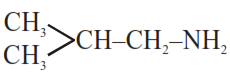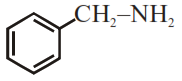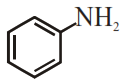# The total number of amines among the following`
Question:

The total number of amines among the following which can be synthesized by Gabriel synthesis is

1.2. $\mathrm{CH}_{3} \mathrm{CH}_{2} \mathrm{NH}_{2}$

3.4.Correct Option: 3,

Solution:

Gabriel phthalimide synthesis is used to prepare $1^{\circ}$ aliphatic/alicyclic amine in common.

Hence amine which can synthesised by Gabriel phthalimide synthesis method is:

(A) $\mathrm{Me}_{2} \mathrm{CH}-\mathrm{CH}_{2}-\mathrm{NH}_{2}$ (B) $\mathrm{CH}_{3} \mathrm{CH}_{2} \mathrm{NH}_{2}$

(C) $\mathrm{Ph}-\mathrm{CH}_{2}-\mathrm{NH}_{2}$